ROOT   Reference Guide
Searching...
No Matches
TSpectrum3 Class Reference

This class contains advanced spectra processing functions.

• Three-dimensional background estimation functions
• Three-dimensional smoothing functions
• Three-dimensional deconvolution functions
• Three-dimensional peak search functions

The algorithms in this class have been published in the following references:

 M.Morhac et al.: Background elimination methods for multidimensional coincidence gamma-ray spectra. Nuclear Instruments and Methods in Physics Research A 401 (1997) 113-132.

 M.Morhac et al.: Efficient one- and two-dimensional Gold deconvolution and its application to gamma-ray spectra decomposition. Nuclear Instruments and Methods in Physics Research A 401 (1997) 385-408.

 M. Morhac et al.: Efficient algorithm of multidimensional deconvolution and its application to nuclear data processing. Digital Signal Processing, Vol. 13, No. 1, (2003), 144-171.

 M.Morhac et al.: Identification of peaks in multidimensional coincidence gamma-ray spectra. Nuclear Instruments and Methods in Research Physics A 443(2000), 108-125.

These NIM papers are also available as Postscript files from:

Definition at line 18 of file TSpectrum3.h.

## Public Types

enum  { kBackIncreasingWindow =0 , kBackDecreasingWindow =1 , kBackSuccessiveFiltering =0 , kBackOneStepFiltering =1 }Public Types inherited from TObject
enum  {
kIsOnHeap = 0x01000000 , kNotDeleted = 0x02000000 , kZombie = 0x04000000 , kInconsistent = 0x08000000 ,
}

enum  { kSingleKey = BIT(0) , kOverwrite = BIT(1) , kWriteDelete = BIT(2) }

enum  EDeprecatedStatusBits { kObjInCanvas = BIT(3) }

enum  EStatusBits {
kCanDelete = BIT(0) , kMustCleanup = BIT(3) , kIsReferenced = BIT(4) , kHasUUID = BIT(5) ,
kCannotPick = BIT(6) , kNoContextMenu = BIT(8) , kInvalidObject = BIT(13)
}

## Public Member Functions

TSpectrum3 ()
Constructor.

TSpectrum3 (Int_t maxpositions, Double_t resolution=1)

virtual ~TSpectrum3 ()
Destructor.

virtual const char * Background (const TH1 *hist, Int_t niter, Option_t *option="goff")
This function calculates background spectrum from source in h.

const char * Background (Double_t ***spectrum, Int_t ssizex, Int_t ssizey, Int_t ssizez, Int_t numberIterationsX, Int_t numberIterationsY, Int_t numberIterationsZ, Int_t direction, Int_t filterType)
This function calculates background spectrum from source spectrum.

const char * Deconvolution (Double_t ***source, const Double_t ***resp, Int_t ssizex, Int_t ssizey, Int_t ssizez, Int_t numberIterations, Int_t numberRepetitions, Double_t boost)
This function calculates deconvolution from source spectrum according to response spectrum The result is placed in the cube pointed by source pointer.

TH1GetHistogram () const

Int_t GetNPeaks () const

Double_tGetPositionX () const

Double_tGetPositionY () const

Double_tGetPositionZ () const

virtual void Print (Option_t *option="") const
Print the array of positions.

virtual Int_t Search (const TH1 *hist, Double_t sigma=2, Option_t *option="goff", Double_t threshold=0.05)
This function searches for peaks in source spectrum in hin The number of found peaks and their positions are written into the members fNpeaks and fPositionX.

Int_t SearchFast (const Double_t ***source, Double_t ***dest, Int_t ssizex, Int_t ssizey, Int_t ssizez, Double_t sigma, Double_t threshold, Bool_t markov, Int_t averWindow)
THREE-DIMENSIONAL CLASSICAL PEAK SEARCH FUNCTION This function searches for peaks in source spectrum using the algorithm based on smoothed second differences.

Int_t SearchHighRes (const Double_t ***source, Double_t ***dest, Int_t ssizex, Int_t ssizey, Int_t ssizez, Double_t sigma, Double_t threshold, Bool_t backgroundRemove, Int_t deconIterations, Bool_t markov, Int_t averWindow)
This function searches for peaks in source spectrum It is based on deconvolution method.

void SetResolution (Double_t resolution=1)
NOT USED resolution: determines resolution of the neighbouring peaks default value is 1 correspond to 3 sigma distance between peaks.

const char * SmoothMarkov (Double_t ***source, Int_t ssizex, Int_t ssizey, Int_t ssizez, Int_t averWindow)
This function calculates smoothed spectrum from source spectrum based on Markov chain method.Public Member Functions inherited from TNamed
TNamed ()

TNamed (const char *name, const char *title)

TNamed (const TNamed &named)
TNamed copy ctor.

TNamed (const TString &name, const TString &title)

virtual ~TNamed ()
TNamed destructor.

virtual void Clear (Option_t *option="")
Set name and title to empty strings ("").

virtual TObjectClone (const char *newname="") const
Make a clone of an object using the Streamer facility.

virtual Int_t Compare (const TObject *obj) const
Compare two TNamed objects.

virtual void Copy (TObject &named) const
Copy this to obj.

virtual void FillBuffer (char *&buffer)
Encode TNamed into output buffer.

virtual const char * GetName () const
Returns name of object.

virtual const char * GetTitle () const
Returns title of object.

virtual ULong_t Hash () const
Return hash value for this object.

virtual Bool_t IsSortable () const

virtual void ls (Option_t *option="") const
List TNamed name and title.

TNamedoperator= (const TNamed &rhs)
TNamed assignment operator.

virtual void SetName (const char *name)
Set the name of the TNamed.

virtual void SetNameTitle (const char *name, const char *title)
Set all the TNamed parameters (name and title).

virtual void SetTitle (const char *title="")
Set the title of the TNamed.

virtual Int_t Sizeof () const
Return size of the TNamed part of the TObject.Public Member Functions inherited from TObject
TObject ()
TObject constructor.

TObject (const TObject &object)
TObject copy ctor.

virtual ~TObject ()
TObject destructor.

void AbstractMethod (const char *method) const
Use this method to implement an "abstract" method that you don't want to leave purely abstract.

Append graphics object to current pad.

virtual void Browse (TBrowser *b)
Browse object. May be overridden for another default action.

ULong_t CheckedHash ()
Check and record whether this class has a consistent Hash/RecursiveRemove setup (*) and then return the regular Hash value for this object.

virtual const char * ClassName () const
Returns name of class to which the object belongs.

virtual void Delete (Option_t *option="")
Delete this object.

virtual Int_t DistancetoPrimitive (Int_t px, Int_t py)
Computes distance from point (px,py) to the object.

virtual void Draw (Option_t *option="")
Default Draw method for all objects.

virtual void DrawClass () const
Draw class inheritance tree of the class to which this object belongs.

virtual TObjectDrawClone (Option_t *option="") const
Draw a clone of this object in the current selected pad for instance with: gROOT->SetSelectedPad(gPad).

virtual void Dump () const
Dump contents of object on stdout.

virtual void Error (const char *method, const char *msgfmt,...) const
Issue error message.

virtual void Execute (const char *method, const char *params, Int_t *error=0)
Execute method on this object with the given parameter string, e.g.

virtual void Execute (TMethod *method, TObjArray *params, Int_t *error=0)
Execute method on this object with parameters stored in the TObjArray.

virtual void ExecuteEvent (Int_t event, Int_t px, Int_t py)
Execute action corresponding to an event at (px,py).

virtual void Fatal (const char *method, const char *msgfmt,...) const
Issue fatal error message.

virtual TObjectFindObject (const char *name) const
Must be redefined in derived classes.

virtual TObjectFindObject (const TObject *obj) const
Must be redefined in derived classes.

virtual Option_tGetDrawOption () const
Get option used by the graphics system to draw this object.

virtual const char * GetIconName () const
Returns mime type name of object.

virtual char * GetObjectInfo (Int_t px, Int_t py) const
Returns string containing info about the object at position (px,py).

virtual Option_tGetOption () const

virtual UInt_t GetUniqueID () const
Return the unique object id.

virtual Bool_t HandleTimer (TTimer *timer)
Execute action in response of a timer timing out.

Bool_t HasInconsistentHash () const
Return true is the type of this object is known to have an inconsistent setup for Hash and RecursiveRemove (i.e.

virtual void Info (const char *method, const char *msgfmt,...) const
Issue info message.

virtual Bool_t InheritsFrom (const char *classname) const
Returns kTRUE if object inherits from class "classname".

virtual Bool_t InheritsFrom (const TClass *cl) const
Returns kTRUE if object inherits from TClass cl.

virtual void Inspect () const
Dump contents of this object in a graphics canvas.

void InvertBit (UInt_t f)

virtual Bool_t IsEqual (const TObject *obj) const
Default equal comparison (objects are equal if they have the same address in memory).

virtual Bool_t IsFolder () const
Returns kTRUE in case object contains browsable objects (like containers or lists of other objects).

R__ALWAYS_INLINE Bool_t IsOnHeap () const

R__ALWAYS_INLINE Bool_t IsZombie () const

void MayNotUse (const char *method) const
Use this method to signal that a method (defined in a base class) may not be called in a derived class (in principle against good design since a child class should not provide less functionality than its parent, however, sometimes it is necessary).

virtual Bool_t Notify ()
This method must be overridden to handle object notification.

void Obsolete (const char *method, const char *asOfVers, const char *removedFromVers) const
Use this method to declare a method obsolete.

void operator delete (void *ptr)
Operator delete.

void operator delete[] (void *ptr)
Operator delete [].

voidoperator new (size_t sz)

voidoperator new (size_t sz, void *vp)

voidoperator new[] (size_t sz)

voidoperator new[] (size_t sz, void *vp)

TObjectoperator= (const TObject &rhs)
TObject assignment operator.

virtual void Paint (Option_t *option="")
This method must be overridden if a class wants to paint itself.

virtual void Pop ()
Pop on object drawn in a pad to the top of the display list.

virtual Int_t Read (const char *name)
Read contents of object with specified name from the current directory.

virtual void RecursiveRemove (TObject *obj)
Recursively remove this object from a list.

void ResetBit (UInt_t f)

virtual void SaveAs (const char *filename="", Option_t *option="") const
Save this object in the file specified by filename.

virtual void SavePrimitive (std::ostream &out, Option_t *option="")
Save a primitive as a C++ statement(s) on output stream "out".

void SetBit (UInt_t f)

void SetBit (UInt_t f, Bool_t set)
Set or unset the user status bits as specified in f.

virtual void SetDrawOption (Option_t *option="")
Set drawing option for object.

virtual void SetUniqueID (UInt_t uid)
Set the unique object id.

virtual void SysError (const char *method, const char *msgfmt,...) const
Issue system error message.

R__ALWAYS_INLINE Bool_t TestBit (UInt_t f) const

Int_t TestBits (UInt_t f) const

virtual void UseCurrentStyle ()
Set current style settings in this object This function is called when either TCanvas::UseCurrentStyle or TROOT::ForceStyle have been invoked.

virtual void Warning (const char *method, const char *msgfmt,...) const
Issue warning message.

virtual Int_t Write (const char *name=0, Int_t option=0, Int_t bufsize=0)
Write this object to the current directory.

virtual Int_t Write (const char *name=0, Int_t option=0, Int_t bufsize=0) const
Write this object to the current directory.

## Protected Attributes

TH1fHistogram
resulting histogram

Int_t fMaxPeaks
Maximum number of peaks to be found.

Int_t fNPeaks
number of peaks found

Double_tfPosition
[fNPeaks] array of current peak positions

Double_tfPositionX
[fNPeaks] X positions of peaks

Double_tfPositionY
[fNPeaks] Y positions of peaks

Double_tfPositionZ
[fNPeaks] Z positions of peaks

Double_t fResolution
NOT USED resolution of the neighboring peaksProtected Attributes inherited from TNamed
TString fName

TString fTitleStatic Public Member Functions inherited from TObject
static Long_t GetDtorOnly ()
Return destructor only flag.

static Bool_t GetObjectStat ()
Get status of object stat flag.

static void SetDtorOnly (void *obj)
Set destructor only flag.

static void SetObjectStat (Bool_t stat)
Turn on/off tracking of objects in the TObjectTable.Protected Types inherited from TObject
enum  { kOnlyPrepStep = BIT(3) }Protected Member Functions inherited from TObject
virtual void DoError (int level, const char *location, const char *fmt, va_list va) const
Interface to ErrorHandler (protected).

void MakeZombie ()

#include <TSpectrum3.h>

Inheritance diagram for TSpectrum3:
[legend]

## ◆ anonymous enum

 anonymous enum
Enumerator
kBackIncreasingWindow
kBackDecreasingWindow
kBackSuccessiveFiltering
kBackOneStepFiltering

Definition at line 30 of file TSpectrum3.h.

## ◆ TSpectrum3() [1/2]

 TSpectrum3::TSpectrum3 ( )

Constructor.

Definition at line 58 of file TSpectrum3.cxx.

## ◆ TSpectrum3() [2/2]

 TSpectrum3::TSpectrum3 ( Int_t maxpositions, Double_t resolution = 1 )
• maxpositions: maximum number of peaks
• resolution: NOT USED determines resolution of the neighbouring peaks default value is 1 correspond to 3 sigma distance between peaks. Higher values allow higher resolution (smaller distance between peaks. May be set later through SetResolution.

Definition at line 80 of file TSpectrum3.cxx.

## ◆ ~TSpectrum3()

 TSpectrum3::~TSpectrum3 ( )
virtual

Destructor.

Definition at line 97 of file TSpectrum3.cxx.

## ◆ Background() [1/2]

 const char * TSpectrum3::Background ( const TH1 * h, Int_t number_of_iterations, Option_t * option = "goff" )
virtual

This function calculates background spectrum from source in h.

The result is placed in the vector pointed by spectrum pointer.

Function parameters:

• spectrum: pointer to the vector of source spectrum
• size: length of spectrum and working space vectors
• number_of_iterations, for details we refer to manual

Definition at line 115 of file TSpectrum3.cxx.

## ◆ Background() [2/2]

 const char * TSpectrum3::Background ( Double_t *** spectrum, Int_t ssizex, Int_t ssizey, Int_t ssizez, Int_t numberIterationsX, Int_t numberIterationsY, Int_t numberIterationsZ, Int_t direction, Int_t filterType )

This function calculates background spectrum from source spectrum.

The result is placed to the array pointed by spectrum pointer.

Function parameters:

• spectrum-pointer to the array of source spectrum
• ssizex-x length of spectrum
• ssizey-y length of spectrum
• ssizez-z length of spectrum
• numberIterationsX-maximal x width of clipping window
• numberIterationsY-maximal y width of clipping window
• numberIterationsZ-maximal z width of clipping window for details we refer to manual
• direction- direction of change of clipping window
• possible values=kBackIncreasingWindow, kBackDecreasingWindow
• filterType-determines the algorithm of the filtering -possible values=kBackSuccessiveFiltering, kBackOneStepFiltering

### Background estimation

Goal: Separation of useful information (peaks) from useless information (background)

• method is based on Sensitive Nonlinear Iterative Peak (SNIP) clipping algorithm 
• there exist two algorithms for the estimation of new value in the channel $$i_1, i_2, i_3$$

#### Algorithm based on Successive Comparisons

It is an extension of one-dimensional SNIP algorithm to another dimension. For details we refer to .

#### Algorithm based on One Step Filtering

The algorithm is analogous to that for 2-dimensional data. For details we refer to TSpectrum2. New value in the estimated channel is calculated as $$a = \nu_{p-1}(i_1, i_2, i_3)$$$\nu_p(i_1, i_2, i_3) = min (a,b)$

where p = 1, 2, ..., number_of_iterations.

#### References:

 C. G Ryan et al.: SNIP, a statistics-sensitive background treatment for the quantitative analysis of PIXE spectra in geoscience applications. NIM, B34 (1988), 396-402./

 M.Morhac, J. Kliman, V. Matouoek, M. Veselsky, I. Turzo.: Background elimination methods for multidimensional gamma-ray spectra. NIM, A401 (1997) 113-132.

Example 1- script Back3.c :Fig. 1 Original three-dimensional gamma-gamma-gamma-ray spectrum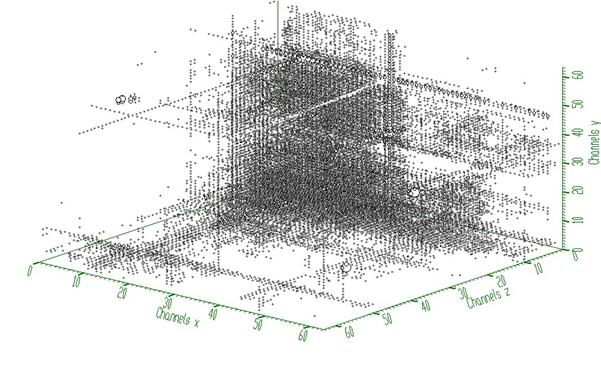Fig. 2 Background estimated from data from Fig. 1 using decreasing clipping window with widths 5, 5, 5 and algorithm based on successive comparisons. The estimate includes not only continuously changing background but also one- and two-dimensional ridges.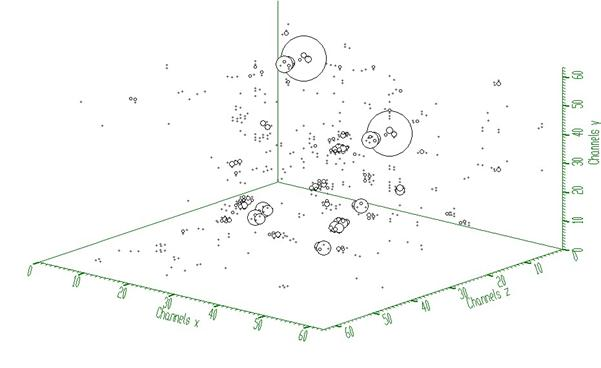Fig. 3 Resulting peaks after subtraction of the estimated background (Fig. 2) from original three-dimensional gamma-gamma-gamma-ray spectrum (Fig. 1).

#### Script:

Example to illustrate the background estimator (class TSpectrum3). To execute this example, do:

root > .x Back3.C

void Back3() {
Int_t i, j, k;
Int_t nbinsx = 64;
Int_t nbinsy = 64;
Int_t nbinsz = 64;
Int_t xmin = 0;
Int_t xmax = nbinsx;
Int_t ymin = 0;
Int_t ymax = nbinsy;
Int_t zmin = 0;
Int_t zmax = nbinsz;
Double_t*** source = new Double_t**[nbinsx];
Double_t*** dest = new Double_t**[nbinsx];
for(i=0;i<nbinsx;i++){
source[i]=new Double_t*[nbinsy];
for(j=0;j<nbinsy;j++)
source[i][j]=new Double_t[nbinsz];
}
for(i=0;i<nbinsx;i++){
dest[i]=new Double_t*[nbinsy];
for(j=0;j<nbinsy;j++)
dest[i][j]=new Double_t[nbinsz];
}
TH3F *back = new TH3F("back","Background estimation",nbinsx,xmin,xmax,nbinsy,ymin,ymax,nbinsz,zmin,zmax);
TFile *f = new TFile("TSpectrum3.root");
back=(TH3F*)f->Get("back;1");
TCanvas *Background = new TCanvas("Background","Estimation of background with decreasing window",10,10,1000,700);
TSpectrum3 *s = new TSpectrum3();
for (i = 0; i < nbinsx; i++){
for (j = 0; j < nbinsy; j++){
for (k = 0; k < nbinsz; k++){
source[i][j][k] = back->GetBinContent(i + 1,j + 1,k + 1);
dest[i][j][k] = back->GetBinContent(i + 1,j + 1,k + 1);
}
}
}
s->Background(dest,nbinsx,nbinsy,nbinsz,5,5,5,s->kBackDecreasingWindow,s->kBackSuccessiveFiltering);
for (i = 0; i < nbinsx; i++){
for (j = 0; j < nbinsy; j++){
for (k = 0; k < nbinsz; k++){
back->SetBinContent(i + 1,j + 1,k + 1, dest[i][j][k]);
}
}
}
FILE *out;
char PATH;
strcpy(PATH,"spectra3/back_output_5ds.spe");
out=fopen(PATH,"wb");
for(i=0;i<nbinsx;i++){
for(j=0;j<nbinsy;j++){
fwrite(dest[i][j], sizeof(dest),nbinsz,out);
}
}
fclose(out);
for (i = 0; i < nbinsx; i++){
for (j = 0; j <nbinsy; j++){
for (k = 0; k <nbinsz; k++){
source[i][j][k] = source[i][j][k] - dest[i][j][k];
}
}
}
for (i = 0; i < nbinsx; i++){
for (j = 0; j < nbinsy; j++){
for (k = 0; k < nbinsz; k++){
back->SetBinContent(i + 1,j + 1,k + 1, source[i][j][k]);
}
}
}
strcpy(PATH,"spectra3/back_peaks_5ds.spe");
out=fopen(PATH,"wb");
for(i=0;i<nbinsx;i++){
for(j=0;j<nbinsy;j++){
fwrite(source[i][j], sizeof(source),nbinsz,out);
}
}
fclose(out);
back->Draw("");
}
#define f(i)
Definition RSha256.hxx:104
double Double_t
Definition RtypesCore.h:59
float xmin
float ymin
float xmax
float ymax
The Canvas class.
Definition TCanvas.h:23
A ROOT file is a suite of consecutive data records (TKey instances) with a well defined format.
Definition TFile.h:54
virtual void Draw(Option_t *option="")
Draw this histogram with options.
Definition TH1.cxx:3073
3-D histogram with a float per channel (see TH1 documentation)}
Definition TH3.h:268
virtual Double_t GetBinContent(Int_t bin) const
Return content of bin number bin.
Definition TH3.h:96
virtual void SetBinContent(Int_t bin, Double_t content)
Set bin content.
Definition TH3.cxx:3339
Definition TSpectrum3.h:18
virtual const char * Background(const TH1 *hist, Int_t niter, Option_t *option="goff")
This function calculates background spectrum from source in h.
@ kBackSuccessiveFiltering
Definition TSpectrum3.h:33
@ kBackDecreasingWindow
Definition TSpectrum3.h:32
TSpectrum3()
Constructor.
#define dest(otri, vertexptr)
Definition triangle.c:1040

Definition at line 384 of file TSpectrum3.cxx.

## ◆ Deconvolution()

 const char * TSpectrum3::Deconvolution ( Double_t *** source, const Double_t *** resp, Int_t ssizex, Int_t ssizey, Int_t ssizez, Int_t numberIterations, Int_t numberRepetitions, Double_t boost )

This function calculates deconvolution from source spectrum according to response spectrum The result is placed in the cube pointed by source pointer.

Function parameters:

• source-pointer to the cube of source spectrum
• resp-pointer to the cube of response spectrum
• ssizex-x length of source and response spectra
• ssizey-y length of source and response spectra
• ssizey-y length of source and response spectra
• numberIterations, for details we refer to manual
• numberRepetitions, for details we refer to manual
• boost, boosting factor, for details we refer to manual

### Deconvolution

Goal: Improvement of the resolution in spectra, decomposition of multiplets

Mathematical formulation of the 3-dimensional convolution system is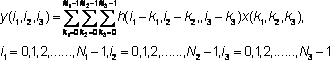where h(i,j,k) is the impulse response function, x, y are input and output fields, respectively, $$N_1, N_2, N3$$, are the lengths of x and h fields

• let us assume that we know the response and the output fields (spectra) of the above given system.
• the deconvolution represents solution of the overdetermined system of linear equations, i.e., the calculation of the field -x.
• from numerical stability point of view the operation of deconvolution is extremely critical (ill-posed problem) as well as time consuming operation.
• the Gold deconvolution algorithm proves to work very well even for 2-dimensional systems. Generalization of the algorithm for 2-dimensional systems was presented in , and for multidimensional systems in .
• for Gold deconvolution algorithm as well as for boosted deconvolution algorithm we refer also to TSpectrum and TSpectrum2

#### References:

 M.Morhac, J. Kliman, V. Matouoek, M. Veselsky, I. Turzo.: Efficient one- and two-dimensional Gold deconvolution and its application to gamma-ray spectra decomposition. NIM, A401 (1997) 385-408.

 Morhac M., Matouoek V., Kliman J., Efficient algorithm of multidimensional deconvolution and its application to nuclear data processing, Digital Signal Processing 13 (2003) 144.

### Example 1 - script Decon.c :

response function (usually peak) should be shifted to the beginning of the coordinate system (see Fig. 1)Fig. 1 Three-dimensional response spectrum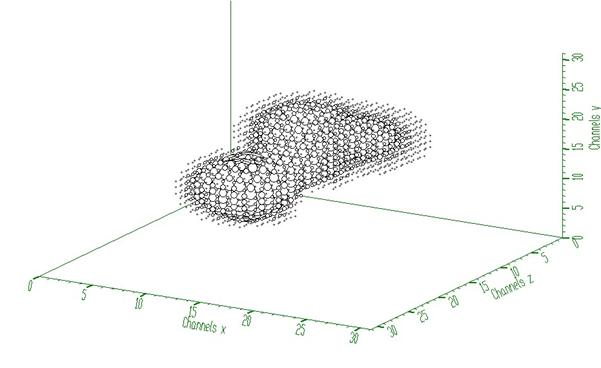Fig. 2 Three-dimensional input spectrum (before deconvolution)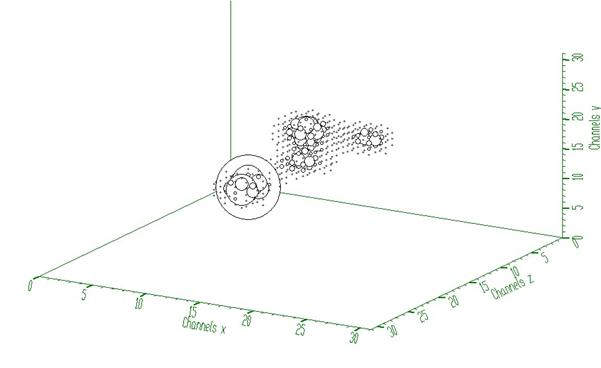Fig. 3 Spectrum from Fig. 2 after deconvolution (100 iterations)

#### Script:

Example to illustrate the Gold deconvolution (class TSpectrum3). To execute this example, do:

root > .x Decon3.C

#include <TSpectrum3>
void Decon3() {
Int_t i, j, k;
Int_t nbinsx = 32;
Int_t nbinsy = 32;
Int_t nbinsz = 32;
Int_t xmin = 0;
Int_t xmax = nbinsx;
Int_t ymin = 0;
Int_t ymax = nbinsy;
Int_t zmin = 0;
Int_t zmax = nbinsz;
Double_t*** source = newDouble_t**[nbinsx];
Double_t*** resp = new Double_t**[nbinsx];
for(i=0;i<nbinsx;i++){
source[i]=new Double_t* [nbinsy];
for(j=0;j<nbinsy;j++)
source[i][j]=new Double_t[nbinsz];
}
for(i=0;i<nbinsx;i++){
resp[i]=new Double_t*[nbinsy];
for(j=0;j<nbinsy;j++)
resp[i][j]=new Double_t[nbinsz];
}
TH3F *decon_in = new TH3F("decon_in","Deconvolution",nbinsx,xmin,xmax,nbinsy,ymin,ymax,nbinsz,zmin,zmax);
TH3F *decon_resp = new TH3F("decon_resp","Deconvolution",nbinsx,xmin,xmax,nbinsy,ymin,ymax,nbinsz,zmin,zmax);
TFile *f = new TFile("TSpectrum3.root");
decon_in=(TH3F*) f->Get("decon_in;1");
decon_resp=(TH3F*) f->Get("decon_resp;1");
TCanvas *Deconvolution = new TCanvas("Deconvolution","Deconvolution of 3-dimensional spectra",10,10,1000,700);
TSpectrum3 *s = new TSpectrum3();
for (i = 0; i < nbinsx; i++){
for (j = 0; j < nbinsy; j++){
for (k = 0; k < nbinsz; k++){
source[i][j][k] = decon_in->GetBinContent(i + 1,j + 1,k + 1);
resp[i][j][k] = decon_resp->GetBinContent(i + 1,j + 1,k + 1);
}
}
}
s->Deconvolution(source,resp,nbinsx,nbinsy,nbinsz,100,1,1);
for (i = 0; i < nbinsx; i++){
for (j = 0; j < nbinsy; j++){
for (k = 0; k < nbinsz; k++){
decon_in->SetBinContent(i + 1,j + 1,k + 1, source[i][j][k]);
}
}
}
decon_in->Draw("");
}
const char * Deconvolution(Double_t ***source, const Double_t ***resp, Int_t ssizex, Int_t ssizey, Int_t ssizez, Int_t numberIterations, Int_t numberRepetitions, Double_t boost)
This function calculates deconvolution from source spectrum according to response spectrum The result...

### Example 2 - script Decon_hr.c :

This example illustrates repeated Gold deconvolution with boosting. After every 10 iterations we apply power function with exponent = 2 to the spectrum given in Fig. 2.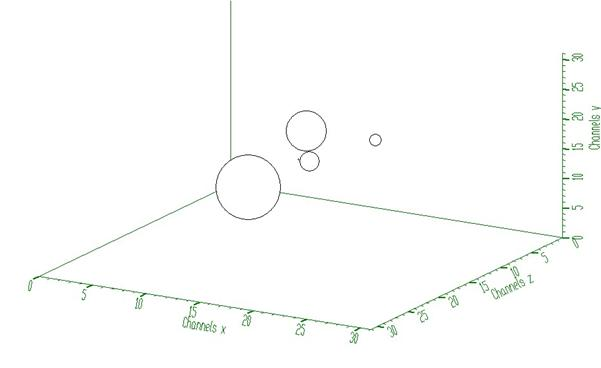Fig. 4 Spectrum from Fig. 2 after boosted deconvolution (10 iterations repeated 10 times). It decomposes completely cluster of peaks from Fig 2.

#### Script:

Example to illustrate the Gold deconvolution (class TSpectrum3). To execute this example, do:

root > .x Decon3_hr.C

void Decon3_hr() {
Int_t i, j, k;
Int_t nbinsx = 32;
Int_t nbinsy = 32;
Int_t nbinsz = 32;
Int_t xmin = 0;
Int_t xmax = nbinsx;
Int_t ymin = 0;
Int_t ymax = nbinsy;
Int_t zmin = 0;
Int_t zmax = nbinsz;
Double_t*** source = new Double_t**[nbinsx];
Double_t*** resp = new Double_t**[nbinsx];
for(i=0;i<nbinsx;i++){
source[i]=new Double_t*[nbinsy];
for(j=0;j<nbinsy;j++)
source[i][j]=new Double_t[nbinsz];
}
for(i=0;i<nbinsx;i++){
resp[i]=new Double_t*[nbinsy];
for(j=0;j<nbinsy;j++)
resp[i][j]=new Double_t[nbinsz];
}
TH3F *decon_in = new TH3F("decon_in","Deconvolution",nbinsx,xmin,xmax,nbinsy,ymin,ymax,nbinsz,zmin,zmax);
TH3F *decon_resp = new TH3F("decon_resp","Deconvolution",nbinsx,xmin,xmax,nbinsy,ymin,ymax,nbinsz,zmin,zmax);
TFile *f = new TFile("TSpectrum3.root");
decon_in=(TH3F*)f->Get("decon_in;1");
decon_resp=(TH3F*)f->Get("decon_resp;1");
TCanvas *Deconvolution = new TCanvas("Deconvolution","High resolution deconvolution of 3-dimensional spectra",10,10,1000,700);
TSpectrum3 *s = new TSpectrum3();
for (i = 0; i < nbinsx; i++){
for (j = 0; j < nbinsy; j++){
for (k = 0; k < nbinsz; k++){
source[i][j][k] = decon_in->GetBinContent(i + 1,j + 1,k + 1);
resp[i][j][k] = decon_resp->GetBinContent(i + 1,j + 1,k + 1);
}
}
}
s->Deconvolution(source,resp,nbinsx,nbinsy,nbinsz,10,10,2);
for (i = 0; i < nbinsx; i++){
for (j = 0; j < nbinsy; j++){
for (k = 0; k < nbinsz; k++){
decon_in->SetBinContent(i + 1,j + 1,k + 1, source[i][j][k]);
}
}
}
decon_in->Draw("");
}

Definition at line 1597 of file TSpectrum3.cxx.

## ◆ GetHistogram()

 TH1 * TSpectrum3::GetHistogram ( ) const
inline

Definition at line 43 of file TSpectrum3.h.

## ◆ GetNPeaks()

 Int_t TSpectrum3::GetNPeaks ( ) const
inline

Definition at line 44 of file TSpectrum3.h.

## ◆ GetPositionX()

 Double_t * TSpectrum3::GetPositionX ( ) const
inline

Definition at line 45 of file TSpectrum3.h.

## ◆ GetPositionY()

 Double_t * TSpectrum3::GetPositionY ( ) const
inline

Definition at line 46 of file TSpectrum3.h.

## ◆ GetPositionZ()

 Double_t * TSpectrum3::GetPositionZ ( ) const
inline

Definition at line 47 of file TSpectrum3.h.

## ◆ Print()

 void TSpectrum3::Print ( Option_t * option = "" ) const
virtual

Print the array of positions.

Reimplemented from TNamed.

Definition at line 126 of file TSpectrum3.cxx.

## ◆ Search()

 Int_t TSpectrum3::Search ( const TH1 * hin, Double_t sigma = 2, Option_t * option = "goff", Double_t threshold = 0.05 )
virtual

This function searches for peaks in source spectrum in hin The number of found peaks and their positions are written into the members fNpeaks and fPositionX.

Function parameters:

• hin: pointer to the histogram of source spectrum
• sigma: sigma of searched peaks, for details we refer to manual Note that sigma is in number of bins
• threshold: (default=0.05) peaks with amplitude less than threshold*highest_peak are discarded.

if option is not equal to "goff" (goff is the default), then a polymarker object is created and added to the list of functions of the histogram. The histogram is drawn with the specified option and the polymarker object drawn on top of the histogram. The polymarker coordinates correspond to the npeaks peaks found in the histogram. A pointer to the polymarker object can be retrieved later via:

TList *functions = hin->GetListOfFunctions();
TPolyMarker *pm = (TPolyMarker*)functions->FindObject("TPolyMarker")
Definition TList.h:44
virtual TObject * FindObject(const char *name) const
Find an object in this list using its name.
Definition TList.cxx:578
A PolyMarker is defined by an array on N points in a 2-D space.
Definition TPolyMarker.h:31

Definition at line 160 of file TSpectrum3.cxx.

## ◆ SearchFast()

 Int_t TSpectrum3::SearchFast ( const Double_t *** source, Double_t *** dest, Int_t ssizex, Int_t ssizey, Int_t ssizez, Double_t sigma, Double_t threshold, Bool_t markov, Int_t averWindow )

THREE-DIMENSIONAL CLASSICAL PEAK SEARCH FUNCTION This function searches for peaks in source spectrum using the algorithm based on smoothed second differences.

Function parameters:

• source-pointer to the matrix of source spectrum
• ssizex-x length of source spectrum
• ssizey-y length of source spectrum
• ssizez-z length of source spectrum
• sigma-sigma of searched peaks, for details we refer to manual
• threshold-threshold value in % for selected peaks, peaks with amplitude less than threshold*highest_peak/100 are ignored, see manual
• markov-logical variable, if it is true, first the source spectrum is replaced by new spectrum calculated using Markov chains method.
• averWindow-averaging window of searched peaks, for details we refer to manual (applies only for Markov method)

Definition at line 3174 of file TSpectrum3.cxx.

## ◆ SearchHighRes()

 Int_t TSpectrum3::SearchHighRes ( const Double_t *** source, Double_t *** dest, Int_t ssizex, Int_t ssizey, Int_t ssizez, Double_t sigma, Double_t threshold, Bool_t backgroundRemove, Int_t deconIterations, Bool_t markov, Int_t averWindow )

This function searches for peaks in source spectrum It is based on deconvolution method.

First the background is removed (if desired), then Markov spectrum is calculated (if desired), then the response function is generated according to given sigma and deconvolution is carried out. It returns number of found peaks.

Function parameters:

• source-pointer to the matrix of source spectrum
• dest-pointer to the matrix of resulting deconvolved spectrum
• ssizex-x length of source spectrum
• ssizey-y length of source spectrum
• ssizez-z length of source spectrum
• sigma-sigma of searched peaks, for details we refer to manual
• threshold-threshold value in % for selected peaks, peaks with amplitude less than threshold*highest_peak/100 are ignored, see manual
• backgroundRemove-logical variable, set if the removal of background before deconvolution is desired
• deconIterations-number of iterations in deconvolution operation
• markov-logical variable, if it is true, first the source spectrum is replaced by new spectrum calculated using Markov chains method.
• averWindow-averaging window of searched peaks, for details we refer to manual (applies only for Markov method)

### Peaks searching

Goal: to identify automatically the peaks in spectrum with the presence of the continuous background, one- and two-fold coincidences (ridges) and statistical fluctuations - noise.

The common problems connected with correct peak identification in three-dimensional coincidence spectra are

• non-sensitivity to noise, i.e., only statistically relevant peaks should be identified
• non-sensitivity of the algorithm to continuous background
• non-sensitivity to one-fold coincidences (coincidences peak - peak - background in all dimensions) and their crossings
• non-sensitivity to two-fold coincidences (coincidences peak - background - background in all dimensions) and their crossings
• ability to identify peaks close to the edges of the spectrum region
• resolution, decomposition of doublets and multiplets. The algorithm should be able to recognise close positioned peaks.

#### References:

 M.A. Mariscotti: A method for identification of peaks in the presence of background and its application to spectrum analysis. NIM 50 (1967), 309-320.

 M.Morhac, J. Kliman, V. Matouoek, M. Veselsky, I. Turzo.:Identification of peaks in multidimensional coincidence gamma-ray spectra. NIM, A443 (2000) 108-125.

 Z.K. Silagadze, A new algorithm for automatic photo-peak searches. NIM A 376 (1996), 451.

### Example of peak searching method

SearchHighRes function provides users with the possibility to vary the input parameters and with the access to the output deconvolved data in the destination spectrum. Based on the output data one can tune the parameters.

#### Example 1 - script Search3.c:Fig. 1 Three-dimensional spectrum with 5 peaks (sigma=2, threshold=5%, 3 iterations steps in the deconvolution)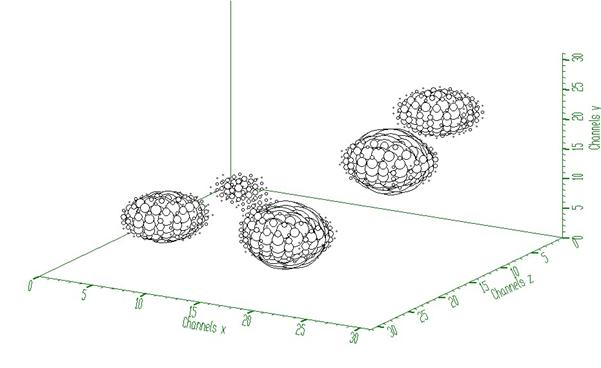Fig. 2 Spectrum from Fig. 1 after background elimination and deconvolution

#### Script:

Example to illustrate high resolution peak searching function (class TSpectrum3). To execute this example, do:

root > .x Search3.C

void Search3() {
Int_t i, j, k, nfound;
Int_t nbinsx = 32;
Int_t nbinsy = 32;
Int_t nbinsz = 32;
Int_t xmin = 0;
Int_t xmax = nbinsx;
Int_t ymin = 0;
Int_t ymax = nbinsy;
Int_t zmin = 0;
Int_t zmax = nbinsz;
Double_t*** source = new Double_t**[nbinsx];
Double_t*** dest = new Double_t**[nbinsx];
for(i=0;i<nbinsx;i++){
source[i]=new Double_t*[nbinsy];
for(j=0;j<nbinsy;j++)
source[i][j]=new Double_t[nbinsz];
}
for(i=0;i<nbinsx;i++){
dest[i]=new Double_t*[nbinsy];
for(j=0;j<nbinsy;j++)
dest[i][j]=new Double_t [nbinsz];
}
TH3F *search = new TH3F("Search","Peak searching",nbinsx,xmin,xmax,nbinsy,ymin,ymax,nbinsz,zmin,zmax);
TFile *f = new TFile("TSpectrum3.root");
search=(TH3F*)f->Get("search2;1");
TCanvas *Search = new TCanvas("Search","Peak searching",10,10,1000,700);
TSpectrum3 *s = new TSpectrum3();
for (i = 0; i < nbinsx; i++){
for (j = 0; j < nbinsy; j++){
for (k = 0; k < nbinsz; k++){
source[i][j][k] = search->GetBinContent(i + 1,j + 1,k + 1);
}
}
}
nfound = s->SearchHighRes(source, dest, nbinsx, nbinsy, nbinsz, 2, 5, kTRUE, 3, kFALSE, 3);
printf("Found %d candidate peaks\n",nfound);
for (i = 0; i < nbinsx; i++){
for (j = 0; j < nbinsy; j++){
for (k = 0; k < nbinsz; k++){
search->SetBinContent(i + 1,j + 1,k + 1, dest[i][j][k]);
}
}
}
Double_t *PosX = new Double_t[nfound];
Double_t *PosY = new Double_t[nfound];
Double_t *PosZ = new Double_t[nfound];
PosX = s->GetPositionX();
PosY = s->GetPositionY();
PosZ = s->GetPositionZ();
for(i=0;i<nfound;i++)
printf("posx= %d, posy= %d, posz=%d\n",(Int_t)(PosX[i]+0.5), (Int_t)(PosY[i]+0.5),(Int_t)(PosZ[i]+0.5));
search->Draw("");
}
int Int_t
Definition RtypesCore.h:45
const Bool_t kFALSE
Definition RtypesCore.h:92
const Bool_t kTRUE
Definition RtypesCore.h:91
Double_t * GetPositionY() const
Definition TSpectrum3.h:46
virtual Int_t Search(const TH1 *hist, Double_t sigma=2, Option_t *option="goff", Double_t threshold=0.05)
This function searches for peaks in source spectrum in hin The number of found peaks and their positi...
Int_t SearchHighRes(const Double_t ***source, Double_t ***dest, Int_t ssizex, Int_t ssizey, Int_t ssizez, Double_t sigma, Double_t threshold, Bool_t backgroundRemove, Int_t deconIterations, Bool_t markov, Int_t averWindow)
This function searches for peaks in source spectrum It is based on deconvolution method.
Double_t * GetPositionX() const
Definition TSpectrum3.h:45
Double_t * GetPositionZ() const
Definition TSpectrum3.h:47

Definition at line 1946 of file TSpectrum3.cxx.

## ◆ SetResolution()

 void TSpectrum3::SetResolution ( Double_t resolution = 1 )

NOT USED resolution: determines resolution of the neighbouring peaks default value is 1 correspond to 3 sigma distance between peaks.

Higher values allow higher resolution (smaller distance between peaks. May be set later through SetResolution.

Definition at line 227 of file TSpectrum3.cxx.

## ◆ SmoothMarkov()

 const char * TSpectrum3::SmoothMarkov ( Double_t *** source, Int_t ssizex, Int_t ssizey, Int_t ssizez, Int_t averWindow )

This function calculates smoothed spectrum from source spectrum based on Markov chain method.

The result is placed in the array pointed by spectrum pointer.

Function parameters:

• source-pointer to the array of source spectrum
• working_space-pointer to the working array
• ssizex-x length of spectrum and working space arrays
• ssizey-y length of spectrum and working space arrays
• ssizey-z length of spectrum and working space arrays
• averWindow-width of averaging smoothing window

### Smoothing

Goal: Suppression of statistical fluctuations the algorithm is based on discrete Markov chain, which has very simple invariant distribution

$U_2 = \frac{p_{1.2}}{p_{2,1}}U_1, U_3 = \frac{p_{2,3}}{p_{3,2}}U_2 U_1, ... , U_n = \frac{p_{n-1,n}}{p_{n,n-1}}U_{n-1} ... U_2 U_1$

$$U_1$$ being defined from the normalization condition $$\sum_{i=1}^{n} U_i = 1$$ n is the length of the smoothed spectrum and

$p_{i,i\pm1} = A_i \sum_{k=1}^{m} exp\left[\frac{y(i\pm k)-y(i)}{y(i\pm k)+y(i)}\right]$

is the probability of the change of the peak position from channel i to the channel i+1. $$A_i$$ is the normalization constant so that $$p_{i,i-1}+p_{i,i+1}=1$$ and m is a width of smoothing window. We have extended this algorithm to three dimensions.

#### Reference:

 Z.K. Silagadze, A new algorithm for automatic photo-peak searches. NIM A 376 (1996), 451-.

### Example 1 - script SmootMarkov3.c :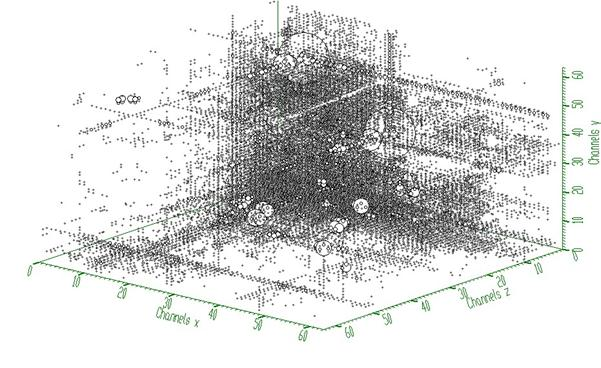Fig. 1 Original noisy spectrum.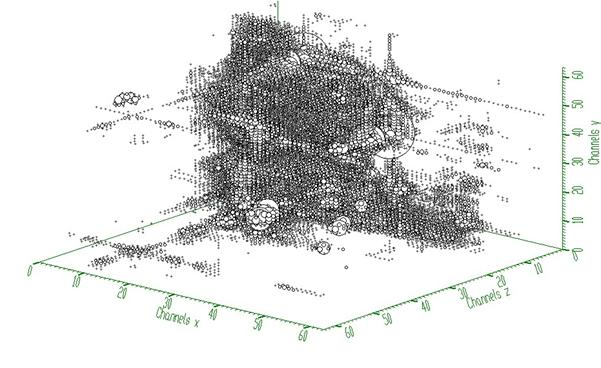Fig. 2 Smoothed spectrum with averaging window m=3.

#### Script:

Example to illustrate the Markov smoothing (class TSpectrum3). To execute this example, do:

root > .x SmoothMarkov3.C

void SmoothMarkov3() {
Int_t i, j, k;
Int_t nbinsx = 64;
Int_t nbinsy = 64;
Int_t nbinsz = 64;
Int_t xmin = 0;
Int_t xmax = nbinsx;
Int_t ymin = 0;
Int_t ymax = nbinsy;
Int_t zmin = 0;
Int_t zmax = nbinsz;
Double_t*** source = new Double_t**[nbinsx];
for(i=0;i<nbinsx;i++){
source[i]=new Double_t*[nbinsy];
for(j=0;j<nbinsy;j++)
source[i][j]=new Double_t[nbinsz];
}
TH3F *sm = new TH3F("Smoothing","Markov smoothing",nbinsx,xmin,xmax,nbinsy,ymin,ymax,nbinsz,zmin,zmax);
TFile *f = new TFile("TSpectrum3.root");
sm=(TH3F*)f->Get("back;1");
TCanvas *Background = new TCanvas("Smoothing","Markov smoothing",10,10,1000,700);
TSpectrum3 *s = new TSpectrum3();
for (i = 0; i < nbinsx; i++){
for (j = 0; j < nbinsy; j++){
for (k = 0; k < nbinsz; k++){
source[i][j][k] = sm->GetBinContent(i + 1,j + 1,k + 1);
}
}
}
s->SmoothMarkov(source,nbinsx,nbinsy,nbinsz,3);
for (i = 0; i < nbinsx; i++){
for (j = 0; j < nbinsy; j++){
for (k = 0; k < nbinsz; k++){
sm->SetBinContent(i + 1,j + 1,k + 1, source[i][j][k]);
}
}
}
sm->Draw("");
}
const char * SmoothMarkov(Double_t ***source, Int_t ssizex, Int_t ssizey, Int_t ssizez, Int_t averWindow)
This function calculates smoothed spectrum from source spectrum based on Markov chain method.

Definition at line 859 of file TSpectrum3.cxx.

## ◆ fHistogram

 TH1* TSpectrum3::fHistogram
protected

resulting histogram

Definition at line 27 of file TSpectrum3.h.

## ◆ fMaxPeaks

 Int_t TSpectrum3::fMaxPeaks
protected

Maximum number of peaks to be found.

Definition at line 20 of file TSpectrum3.h.

## ◆ fNPeaks

 Int_t TSpectrum3::fNPeaks
protected

number of peaks found

Definition at line 21 of file TSpectrum3.h.

## ◆ fPosition

 Double_t* TSpectrum3::fPosition
protected

[fNPeaks] array of current peak positions

Definition at line 22 of file TSpectrum3.h.

## ◆ fPositionX

 Double_t* TSpectrum3::fPositionX
protected

[fNPeaks] X positions of peaks

Definition at line 23 of file TSpectrum3.h.

## ◆ fPositionY

 Double_t* TSpectrum3::fPositionY
protected

[fNPeaks] Y positions of peaks

Definition at line 24 of file TSpectrum3.h.

## ◆ fPositionZ

 Double_t* TSpectrum3::fPositionZ
protected

[fNPeaks] Z positions of peaks

Definition at line 25 of file TSpectrum3.h.

## ◆ fResolution

 Double_t TSpectrum3::fResolution
protected

NOT USED resolution of the neighboring peaks

Definition at line 26 of file TSpectrum3.h.

Libraries for TSpectrum3:[legend]

The documentation for this class was generated from the following files: